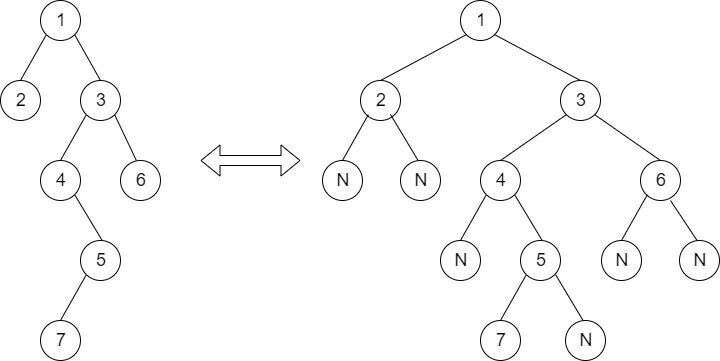Timer is Running

Height of Spiral Tree
##### Submissions: 5390   Accuracy: 41.56%   Difficulty: Easy   Marks: 2
Associated Course(s):   Amazon SDE Test Series

Given a special Binary Tree whose leaf nodes are connected to form a circular doubly linked list. Find the height of this special Binary Tree.

Input:
First line of input contains the number of test cases T. For each test case, there will be only a single line of input which is a string representing the tree as described below:

1. The values in the string are in the order of level order traversal of the tree where, numbers denotes node values, and a character “N” denotes NULL child.

2. For example:For the above tree, the string will be: 1 2 3 N N 4 6 N 5 N N 7 N

Output:
For each testcase in new line, print the height of the tree.

You don't need to read input or print anything. Your task is to complete the function findTreeHeight() which takes the root of the special Binary Tree as its input and returns the Height of this special Binary Tree.
In a special Binary Tree, the leaf nodes form a circular doubly linked list.
For Example:

1
/   \
2    3
/  \
4  5
/

In the above binary tree, 6, 5 and 3 are leaf nodes and they form a circular doubly linked list. Here, the left pointer of leaf node will act as a previous pointer of circular doubly linked list and its right pointer will act as next pointer of circular doubly linked list.

Expected Time Complexity: O(Height of the Tree).
Expected Auxiliary Space: O(Height of the Tree).

Constraints:
1 <= T <= 100
1 <= N <= 104

Example:
Input:

2
1 2 3 4
1 2 3 4 5 N N 6

Output:
3
4

Explanation:
Testcase 1:
There are 3 edges and 4 nodes in spiral tree where leaf nodes 4 and 3 are connected in circular doubly linked list form. Thus the height of spiral tree is 3.

#### ** For More Input/Output Examples Use 'Expected Output' option **

Contributor: Harshit Sidhwa
Author: harshitsidhwa

If you have purchased any course from GeeksforGeeks then please ask your doubt on course discussion forum. You will get quick replies from GFG Moderators there.